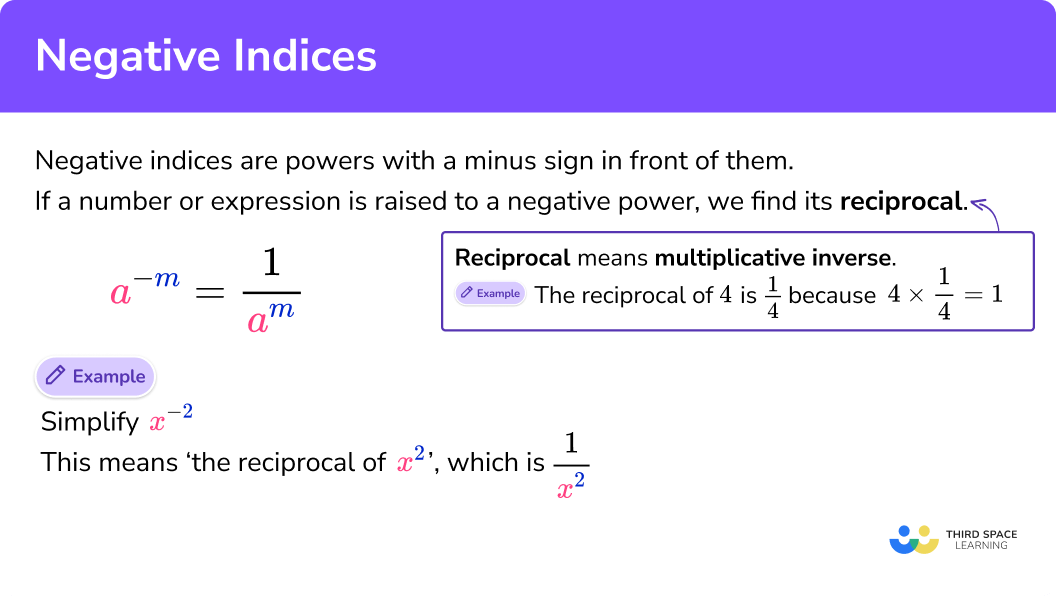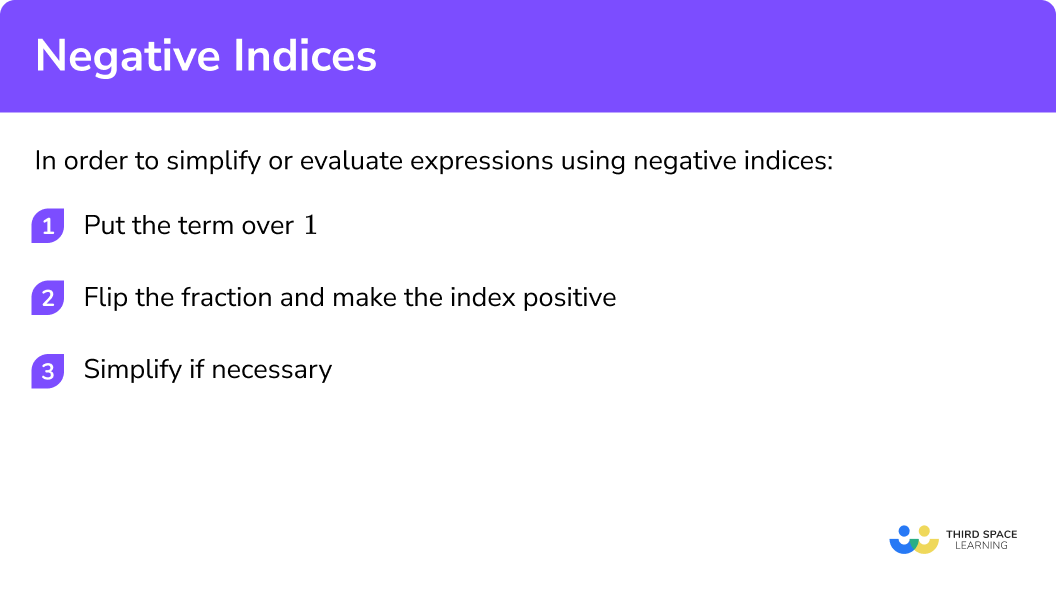GCSE Maths Algebra Law Of Indices

Negative Indices

# Negative Indices

Here we will learn how to simplify and evaluate with negative indices for GCSE & iGCSE maths (Edexcel, AQA and OCR).

Look out for the laws of indices worksheets and exam questions at the end.

## What are negative indices?

Negative indices are powers (also called exponents) with a minus sign in front of them.

E.g.

$x^{-2}$
$3^{-4}$
$2b^{-\frac{1}{2}}$

We get negative indices by dividing two terms with the same base where the first term is raised to a power that is smaller than the power that the second term is raised to.

E.g.

$x^{3} \div x^{4}=\frac{x \times x \times x}{x \times x \times x \times x}$

When we cancel the common factors of x

$\frac{\not x \times \not x \times \not x}{\not x \times \not x \times \not x \times x}$

We are left with

$\frac{1}{x}$

But using the division law of indices we know that

$x^{3} \div x^{4}=x^{3-4}=x^{-1}$

So,

$x^{-1}=\frac{1}{x}$

### What are negative indices?## Related lessons on laws of indices

Negative indices is part of our series of lessons to support revision on laws of indices. You may find it helpful to start with the main laws of indices lesson for a summary of what to expect, or use the step by step guides below for further detail on individual topics. Other lessons in this series include:

## How to use negative indices

To make the negative index positive we put the term over 1 and flip it.

It is known as finding the reciprocal of the term.

E.g.

$x^{-2}=\frac{x^{-2}}{1}=\frac{1}{x^{2}}$
$\frac{1}{x^{2}}$

is the same as

$x^{-2}$

E.g.

$2^{-3}=\frac{2^{-3}}{1}=\frac{1}{2^{3}}=\frac{1}{8}$

Negative exponents will often be used in conjunction with other index laws, including the division, brackets and multiplication laws.

### Explain how to use negative indices## Negative indices examples

### Example 1: no coefficient in front of the base

$a^{-4}$

1. Put the term from the question over 1.

$\frac{a^{-4}}{1}$

2 Flip and change the power from -4 to +4.

$\frac{1}{a^{4}}$

### Example 2: with a coefficient in front of base

$(10 a)^{-3}$

Notice how the index affects the entire bracket.

Put the term over 1.

Flip and change the power -3 to +3.

Simplify the denominator.

### Example 3: with a coefficient in front of base

$3b^{-2}$

Notice how the index only affects the variable b.

Put the term over 1.

Flip and change the power -2 to +2.

### Example 4: with fractional exponents

When dealing with fractions it is easier to skip to step 2.

Evaluate

$\left(\frac{4}{3}\right)^{-2}$

Flip and change the power -2 to +2.

Simplify the numerator and denominator.

### Common misconceptions

• Confusing integer and fractional powers

Raising a term to the power of 2 means we square it.

E.g.

$a^{2}=a \times a$

Raising a term to the power of ½ means we find the square root of it.

E.g.

$a^{\frac{1}{2}}=\pm \sqrt{a}$

Raising a term to the power of 3 means we cube it.

E.g.

$a^{3}=a \times a \times a$

Raising a term to the power of ⅓ means we find the cube root of it.

E.g.

$a^{\frac{1}{3}}=\sqrt{a}$

• Indices, powers or exponents

Indices can also be called powers or exponents.

• Not turning a negative index into a positive index when flipping the term

• Making a mistake when writing one over a fraction

E.g

When we find the reciprocal of

$\left(\frac{2}{3}\right)^{-2}$

we can follow the steps as outlined above

1 Put the term over 1

$\frac{\left(\frac{2}{3}\right)^{-2}}{1}$

2 Flip and change the power -2 to +2

$\frac{1}{\left(\frac{2}{3}\right)^{2}}$

3 Simplify the denominator

$\frac{1}{\left(\frac{2}{3}\right)^{2}}=\frac{1}{\frac{2^{2}}{3^{2}}}=\frac{1}{\frac{4}{9}}$

However, the fraction needs to be simplified further.

$\frac{1}{\frac{4}{9}}=1 \div \frac{4}{9}=1 \times \frac{9}{4}=\frac{9}{4}$

This is why when dealing with fractions it is easier to skip to step 2 and just flip the fraction:

$\left(\frac{2}{3}\right)^{-2}=\left(\frac{3}{2}\right)^{2}=\frac{3^{2}}{2^{2}}=\frac{9}{4}$

### Practice negative indices questions

x^{-5}

x^{5}\frac{1}{x^{-5}}\frac{1}{x^{5}}\frac{1}{5x}The negative index number means we need to find the reciprocal, so

x^{-5}=\frac{1}{x^{5}}

(2 b)^{-4}

16b^{-4}\frac{1}{2 b^{4}}\frac{1}{8 b^{4}}\frac{1}{16 b^{4}}The negative index number means we need to find the reciprocal, so

\begin{aligned} &a(2 b)^{-4}\\ &=\frac{1}{(2b)^{4}}\\ &=\frac{1}{2b\times2b\times2b\times2b}\\ &=\frac{1}{16 b^{4}} \end{aligned}

3 b^{-3}

\frac{1}{b^{3}}\frac{3}{b^{3}}\frac{3}{3b}\frac{1}{3b}The negative index number means we need to find the reciprocal, so

3 b^{-3}=\frac{3}{b^{3}}

\left(\frac{5}{8}\right)^{-1}

-\frac{8}{5}\frac{8}{5}-\frac{3}{5}\frac{3}{5}The negative index number means we need to find the reciprocal, which means inverting the fraction, so

\left(\frac{5}{8}\right)^{-1}=\frac{8}{5}

### Negative indices GCSE questions

1. Evaluate

9^{-2}

(1 mark)

\frac{1}{81}

(1)

2. Evaluate

\left(\frac{4}{5}\right)^{-2}

(2 marks)

\left(\frac{5}{4}\right)^{2}

(1)

\frac{25}{16}

(1)

3. Evaluate

\left(\frac{8}{125}\right)^{-\frac{2}{3}}

(3 marks)

\left(\sqrt{\frac{125}{8}}\right)^{2}

(1)

\left(\frac{5}{2}\right)^{2}

(1)

\frac{25}{4}=6.25

(1)

## Learning checklist

You have now learned how to:

• Simplify expressions involving the laws of indices
• Calculate with roots, and with integer and fractional indices

## Still stuck?

Prepare your KS4 students for maths GCSEs success with Third Space Learning. Weekly online one to one GCSE maths revision lessons delivered by expert maths tutors.

Find out more about our GCSE maths tuition programme.